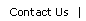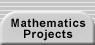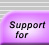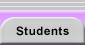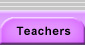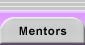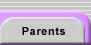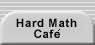# Iteration

Iteration is the repeated application of a function or process in which the output of each step is used as the input for the next iteration. Iteration is an important tool for solving problems (e.g., Newton's method) as well as a subject of investigation (e.g., Julia sets). Any function that has the same type of mathematical object for both its argument and result can be iterated. Examples below include functions iterated on numbers, functions, and geometric figures.

### Examples of problems involving iteration.

The Patterns in Polynomials research project.

Candy for Everyone (iterated sharing).

Integer Iterations on a Circle (iterated arithmetic).

See Iteration (and the other sections) in The Chaos Hypertextbook.

See Newton’s Method at the Visual Calculus site.

Midpoint Triangles – Make the midpoints of the sides of a triangle (A0B0C0) the vertices of a new triangle (A1B1C1). Repeat this process with each new triangle formed. Where in the original triangle does this sequence of triangles head?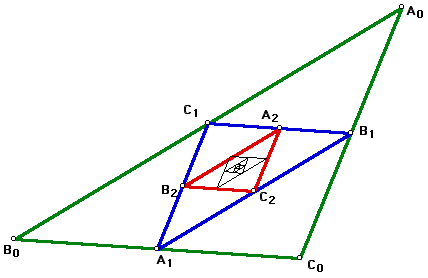Translations of mathematical formulas for web display were created by tex4ht. © Copyright 2003 Education Development Center, Inc. (EDC)